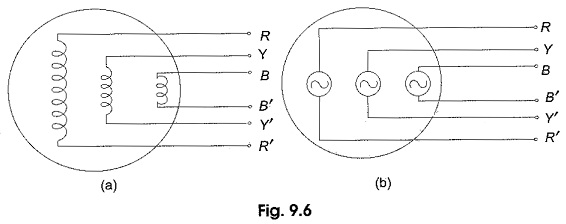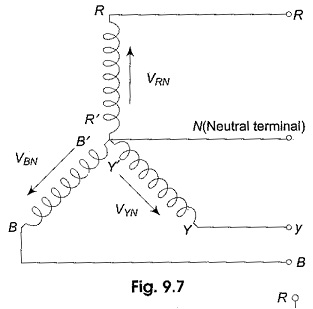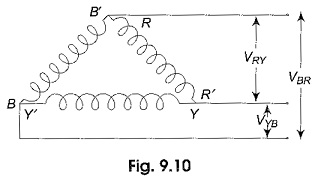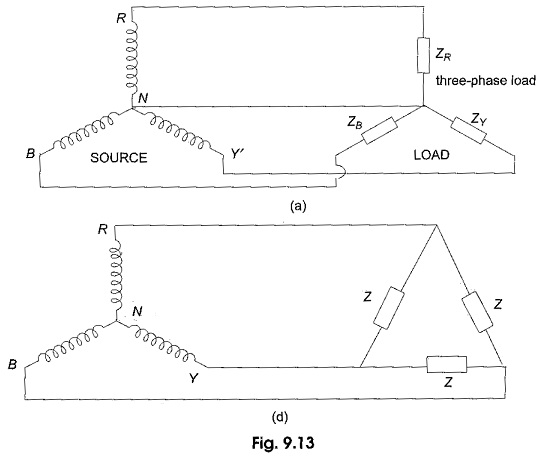## Inter Connection of Three Phase Source and Loads:

Inter Connection of Three Phase Source and Loads consists of for Parts, Namely

• Inter Connection of Three Phase Source
• Wye or Star-Connection
• Delta or Mesh-connection
• Interconnection of Loads

(a) Inter Connection of Three Phase Source:

In a three-phase alternator, there are three independent phase windings or coils. Each phase or coil has two terminals, viz. start and finish. The end connections of the three sets of the coils may be brought out of the machine, to form three separate single phase sources to feed three individual circuits as shown in Fig. 9.6 (a and b).The coils are inter-connected to form a wye (Y) or delta (Δ) connected three-phase system to achieve economy and to reduce the number of conductors, and thereby, the complexity in the circuit. The Three Phase Source so obtained serve all the functions of the three separate single phase sources.

(b) Wye or Star-Connection:

In this connection, similar ends (start or finish) of the Three Phase Source are joined together within the alternator as shown in Fig. 9.7. The common terminal so formed is referred to as the neutral point (N), or neutral terminal. Three lines are run from the other free ends (R, Y, B) to feed power to the three-phase load.Figure 9.7 represents a three-phase, four-wire, star-connected system. The terminals R, Y and B are called the line terminals of the source. The voltage between any line and the neutral point is called the phase voltage (VRN, VYN and VBN), while the voltage between any two lines is called the line voltage (VRY, VYB and VBR). The currents flowing through the phases are called the phase currents, while those flowing in the lines are called the line currents. If the neutral wire is not available for external connection, the system is called a three-phase, three-wire, star-connected system. The system so formed will supply equal line voltages displaced 120° from one another and acting simultaneously in the circuit like three independent single phase sources in the same frame of a three-phase alternator.

(c) Delta or Mesh-connection:

In this method of connection the dissimilar ends of the windings are joined together, i.e. R’ is connected to Y, Y’ to B and B’ to R as shown in Fig. 9.10.The three line conductors are taken from the three junctions of the mesh or delta connection to feed the three-phase load. This constitutes a three-phase, three-wire, delta-connected system. Here there is no common terminal; only three line voltages VRY, VYB and VBR are available.

These line voltages are also referred to as phase voltages in the delta-connected system. When the sources are connected in delta, loads can be connected only across the three line terminals, R, Y and B. In general, a three-phase source, star or delta, can be either balanced or unbalanced. A balanced Three Phase Source is one in which the three individual sources have equal magnitude, with 120° phase difference.

(d) Interconnection of Loads:

The primary question in a star or delta-connected three-phase supply is how to apply the load to the three-phase supply. An impedance, or load, connected across any two terminals of an active network (source) will draw power from the source, and is called a single phase load. Like alternator phase windings, load can also be connected across any two terminals, or between line and neutral terminal (if the source is star-connected). Usually the three-phase load impedances are connected in star or delta formation, and then connected to the three-phase source as shown in Fig. 9.13.Figure 9.13(a) represents the typical inter-connections of loads and sources in a three-phase star system, and is of a three-phase four wire system. A three-phase star connected load is connected to a three-phase star-connected source, terminal to terminal, and both the neutrals are joined with a fourth wire. Figure 9.13(b) is a three-phase, three-wire system. A three-phase, delta-connected load is connected to a three-phase star-connected source, terminal to terminal, as shown in Fig. 9.13(b). When either source or load, or both are connected in delta, only three wires will suffice to connect the load to source.

Just as in the case of a Three Phase Source, a three-phase load can be either balanced or unbalanced. A balanced three-phase load is one in which all the branches have identical impedances, i.e. each impedance has the same magnitude and phase angle. The resistive and reactive components of each phase are equal. Any load which does not satisfy the above requirements is said to be an unbalanced load.